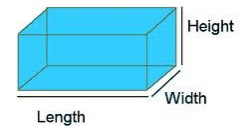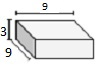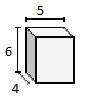# Volume of Rectangular Prism

## Prism

A prism is a solid bounded by a number of plane faces; its two faces, called the ends or bases, are congruent parallel plane polygons and other faces, called the side faces (or lateral faces), and are rectangles.

## Rectangular Prism

In a rectangular prism the bases or ends are rectangular faces and the lateral faces or side faces are also rectangles.## Formula for the volume of a rectangular prism

The volume of a rectangular prism of length l, width w and height h is given by the formula

The product of the length and width of the base of the rectangular prism gives the area of the base A = l × w

The volume of the rectangular prism is given by the product of area of base A and the height h of the prism.

Volume V = A × h = l × w × h cubic units

Find the volume of the given rectangular prism in cubic cm.### Solution

Step 1:

Volume of rectangular prism = l × w × h cubic units.

Step 2:

l = 9 cm; w = 3 cm; h = 9 cm

Volume of given prism = 9 × 3 × 9

= 243 cubic cm.

Find the volume of the given rectangular prism in cubic cm.### Solution

Step 1:

Volume of rectangular prism = l × w × h cubic units

Step 2:

l = 5 cm; w = 6 cm; h = 4 cm

Volume of given prism = 5 × 6 × 4

= 120 cubic cm.